Next: Bibliography Up: thesis Previous: TOTAL ESCAPE PROBABILITY FOR   Contents

# FINDING THE SHORTEST AND THE FASTEST PATHS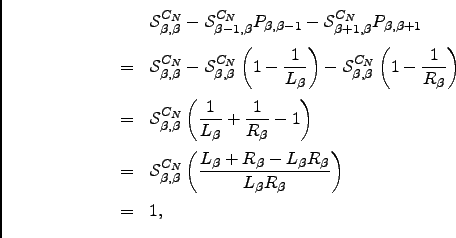Techniques for finding the shortest and the fastest pathways can be useful during sampling for pathways, in post-processing and analysis of DPS databases, and in various optimisations of pathway ensembles [290,291,192,10,8]. Given a digraph representation of a connected database of minima and transition states it is possible to identify the shortest path between any two minima using a breadth-first search algorithm , which runs in linear time. The shortest path, however, is not necessarily the fastest. Recently Evans suggested using Dijkstra's algorithm  for finding the path with the largest non-recrossing DPS rate . It was pointed out by Carr, however, that the weight function used in Reference Evans03 is not positively defined, and the use of the Bellman-Ford-Moore (BFM) algorithm [292,294,293] was suggested instead . Because Dijkstra's algorithm scales better than BFM algorithm (see Figure E.1) we describe below a weight function that enables us to use the Dijkstra's algorithm for finding the fastest path.

As detailed in Section 4.1.1, within a digraph representation of a coarse-grained PES each minimum is represented by a single node and each transition state is represented by two oppositely directed edges. Here we ignore degenerate rearrangements since they do not affect the rates, and for cases where more than one transition state links the same pair of minima only the one with the fastest forward and backward rates is used. Adopting the non-recrossing DPS rate definition from Equation 4.11 we can associate forward and backward rate constants(E.1)

with a pathway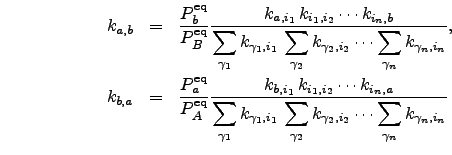.

For a detailed description of Dijkstra and BFM algorithms we refer the reader to Reference CormenLRS01. A suitable choice of edge weight function to be used with either of the shortest path algorithms must be made. We define the weight of each directed edge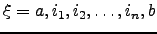as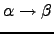(E.2)

Note that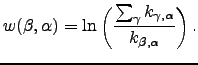and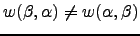. Since the weight is non-negative it is possible to apply Dijkstra's algorithm to find the fastest pathway .

It makes sense to include only minima with more than one connection when searching for the fastest pathway. In our calculations we first iteratively identified a connected subset of minima with the number of connections greater than one, and then run the above algorithm for this subset.

To find the fastest path connecting minima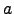and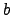in the direction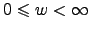it is necessary to solve either a single-source shortest paths problem with minimumchosen as a source, or a single destination shortest paths problem with minimumchosen as the destination. In the latter case all the edges in the graph need to be reversed, which can be accomplished by simply swapping the arguments to the weight function in Equation E.2. This reversal is possible because our graph is a symmetric digraph, i.e. if there is an edge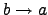then there is also an edge.

A useful check for correct implementation of this algorithm is to verify that the weight of the shortest path from minimumto minimum,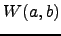, is related to the rate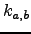calculated for that pathway by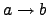(E.3)

The BFM algorithm runs in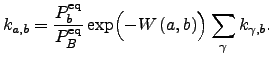time while Dijkstra's algorithm as described in Reference dijkstra59 scales as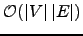. Although both algorithms appear to have similar (quadratic) asymptotic complexity for the case of sparse graphs (e.g.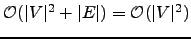) the scaling prefactor is smaller for Dijkstra's algorithm. In addition, for sparse graphs with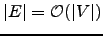an implementation of Dijkstra's algorithm that utilises a priority queue of some sort can improve the asymptotic bounds further. Priority queues based on binary min-heap and Fibonacci heap  result in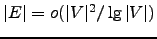and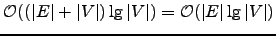scaling of the algorithm, respectively . In the present work we have used a priority queue based on Fibonacci heap. A performance comparison of our implementations of BFM and static Dijkstra's algorithms is shown in Figure E.1.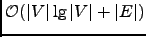Since knowledge of all the pathways on a complicated PES is out of the question a representative sample must be obtained to properly describe the property of interest. It is unclear what is the best strategy of evolving the pathway database if the proper description of kinetics is sought, as sampling techniques may introduce a bias towards pathways of particular type.

In previous DPS studies a set of perturbations was applied to the fastest known path to generate new ones [9,10,8]. New pathways were constructed from a subset of stationary points featured in the pathway being perturbed, and from these that were found as a result of perturbation. Because building the shortest paths tree with Dijkstra's algorithm is relatively cheap it is possible to analyse the whole database for the presence of new faster pathways rather than just the subset. Results for an example application are presented in Figure E.2.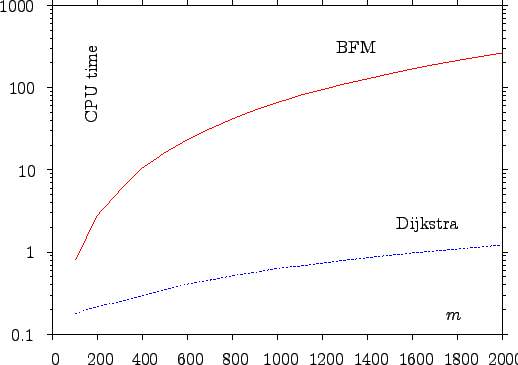After a set of perturbations was applied to the fastest currently known pathway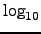Dijkstra's algorithm was used to solve the single-source shortest paths problem to build the shortest paths tree rooted at minimum. For path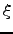the set of perturbations constituted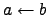attempts to shortcut the path, where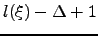is a shortcut parameter,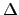.

Each attempt to shortcut the path was realised as a single connect run (see Chapter 2) seeded with two minima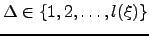separated by a gap of lengthalong the path. If the fastest pathfound by Dijkstra's algorithm was different (not necessarily new) from the one that was currently being perturbed the iteration was completed, this pathway was perturbed in the next iteration and parameterwas reset to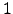. Otherwise,was incremented by one, unless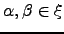in which case the calculation was terminated.Next: Bibliography Up: thesis Previous: TOTAL ESCAPE PROBABILITY FOR   Contents
Semen A Trygubenko 2006-04-10